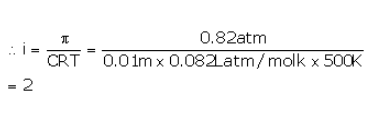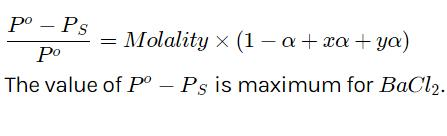Courses

# Test: Association And Dissociation

## 10 Questions MCQ Test Chemistry Class 12 | Test: Association And Dissociation

Description
This mock test of Test: Association And Dissociation for Class 12 helps you for every Class 12 entrance exam. This contains 10 Multiple Choice Questions for Class 12 Test: Association And Dissociation (mcq) to study with solutions a complete question bank. The solved questions answers in this Test: Association And Dissociation quiz give you a good mix of easy questions and tough questions. Class 12 students definitely take this Test: Association And Dissociation exercise for a better result in the exam. You can find other Test: Association And Dissociation extra questions, long questions & short questions for Class 12 on EduRev as well by searching above.
QUESTION: 1

### The osmotic pressure of a 0.010 M MgSO4 solution at 500 K is 0.82 atm. Calculate , the van’t Hoff factor, for this MgSO4 solution.

Solution:QUESTION: 2

### Benzoic acid dissolved in benzene will show a molecular weight of

Solution:

In benzene, benzoic acid exist as dimer. So the molecular weight of benzoic acid gets doubled.

(C6​H5​COOH)2​=2×122=244 units .

QUESTION: 3

### Molar mass that is either lower or higher than expected or normal molar mass is called as

Solution:
QUESTION: 4

0.01 M solution of common salt (NaCl) and (CH3COOH ) is taken. If their osmotic pressures are p1and p2 resp., what will be the correct statement correlating their osmotic pressures?

Solution:
QUESTION: 5

If α is the degree of dissociation of Na2SO4, the vant Hoff’s factor (i) used for calculating the molecular mass is

Solution:
QUESTION: 6

Lowering of vapour pressure is highest for

Solution:QUESTION: 7

Increasing the temperature of solution of a weak electrolyte will cause

Solution:
QUESTION: 8

What is the freezing point of 0.1 m glucose solution in water? For water Kf is 1.86 K kg/mol?

Solution:
QUESTION: 9

van’t Hoff factor is denoted by letter

Solution:
QUESTION: 10

The ratio of osmotic pressure of a 1:1 electrolyte AB to that of a non electrolyte solute of same concentration is

Solution: